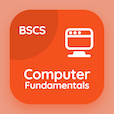Online CS Degree Courses

Database Management System Quizzes

Database Management System Quiz - Complete

Study Introduction to Data Modeling Multiple Choice Questions and Answers (MCQs), introduction to data modeling quiz answers PDF, test 83 to download Database Management System book. Data Modeling Entity Relationship Model MCQ trivia questions, introduction to data modeling Multiple Choice Questions (MCQ Quiz) for online college degrees. Introduction to Data Modeling App Download: knowledge representation and ontology, normalization: second normal form, database constraints and relational schemas, impedance mismatch, introduction to data modeling test prep for 2 year computer science degree.

"The Universal Modeling Language in database management system is classified as" Quiz PDF: introduction to data modeling book with choices query modeling methodology, object modeling methodology, entity modeling methodology, and attribute modeling methodology to learn online certificate courses. Learn data modeling entity relationship model questions and answers to improve problem solving skills for computer and information science.

## Introduction to Data Modeling MCQ Questions

MCQ: The Universal Modeling Language in database management system is classified as

object modeling methodology
query modeling methodology
entity modeling methodology
attribute modeling methodology

MCQ: The problem which arises because of the differences between model of programming language and model of database is classified as

modeling mismatch
referential mismatch
dependence mismatch
impedance mismatch

MCQ: The basic operations that can be performed on relations are

deletion
insertion
modification
all of above

MCQ: The normalization form which is based on the transitive dependency is classified as

first normal form
second normal form
fourth normal form
third normal form

MCQ: The inverse process of classification which focuses on specific examination of different entities of classes is called

identification
exception abstract
classification
instantiation

### More Quizzes from Database Management System Book